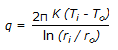# Test: Heat Transfer - 8

## 25 Questions MCQ Test Heat Transfer | Test: Heat Transfer - 8

Description
Attempt Test: Heat Transfer - 8 | 25 questions in 25 minutes | Mock test for Chemical Engineering preparation | Free important questions MCQ to study Heat Transfer for Chemical Engineering Exam | Download free PDF with solutions
QUESTION: 1

Solution:
QUESTION: 2

Solution:
QUESTION: 3

### Rate of heat transfer by vaporisation from pools of water is affected by the

Solution:
QUESTION: 4

Multiple effect evaporators are commonly used in the manufacture of
P. Paper Q. Superphosphate
R. Sugar S. Fats

Solution:
QUESTION: 5

Which of the following has the highest thermal conductivity ?

Solution:
QUESTION: 6

Which of the following is unimportant in forced convection ?

Solution:
QUESTION: 7

Steam is to be condensed in a shell and tube heat exchanger, 5 m long with a shell diameter of 1 m. Cooling water is to be used for removing the heat. Heat transfer co-efficient for the cooling water, whether on shell side or tube side is the same. The best arrangement is

Solution:
QUESTION: 8

In a multiple effect evaporator, the effect of boiling point elevation is to

Solution:
QUESTION: 9

The rate of heat transfer through a pipe wall is given by,. For cylinder of very thin wall, q can be approximated by

Solution:
QUESTION: 10

Dropwise condensation occurs on __________ surfaces.

Solution:
QUESTION: 11

For specified tube outside diameter, higher BWG means higher

Solution:
QUESTION: 12

Pick out the wrong statement.

Solution:
QUESTION: 13

In case of a vertical tube evaporator, with increase in the liquor level, the __________ is increased.

Solution:
QUESTION: 14

Electromagnetic radiations propagate in vacuum with a velocity of __________ metre/second.

Solution:
QUESTION: 15

Heat flux is the time rate of heat transfer per unit

Solution:
QUESTION: 16

Film boiling occurs at __________ pressure.

Solution:
QUESTION: 17

Heat flux through several resistances in series in analogous to the current flowing through several

Solution:
QUESTION: 18

Extended heat transfer surface like fins are used to increase the heat transfer rate. Fin efficiency is defined as the ratio of heat transferred across the fin surface to the theoretical heat transfer across an equal area held at the

Solution:
QUESTION: 19

Vacuum is generally maintained in the vapour space of an evaporator mainly to

Solution:
QUESTION: 20

Double pipe heat exchangers are used

Solution:
QUESTION: 21

Nusselt number (for forced convection heat transfer) is a function of the __________ number.

Solution:
QUESTION: 22

Pick out the wrong statement.

Solution:
QUESTION: 23

Which type of heat exchanger is preferred for heavy heat loads ?

Solution:
QUESTION: 24

Pick out the wrong statement.

Solution:
QUESTION: 25

While the total emissivity of a perfect black body is unity, the same for a real body is

Solution:Use Code STAYHOME200 and get INR 200 additional OFF Use Coupon Code